# ILP Part 33 — Lexicographical comparisons

This is the thirty third part of the ILP series. For your convenience you can find other parts in the table of contents in Part 1 – Boolean algebra

Hi. Today we are going to implement lexicographical comparisons using ILP. Let’s begin!

# Introduction

Let’s assume that we want to solve the following: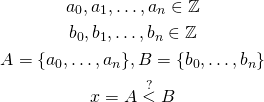So we have two vectors of integer variables and we want to answer the question whether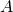is less than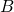. We define “less than” here in usual way, e.g., whether there exists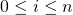for which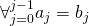and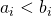.

# Naive implementation

We could implement this naively, e.g., perform all necessary comparisons and come up with the solution using conditions: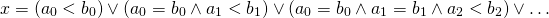This is slow and inefficient. Forelements in vector we haveclauses and each clause contains up toconditions which sums up to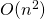conditions. We can do better.

# Better implementation using hashing

We can perform faster comparison using hashes. First, let’s assume that we can operate on numbers up to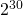and that for now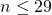. We can do the following: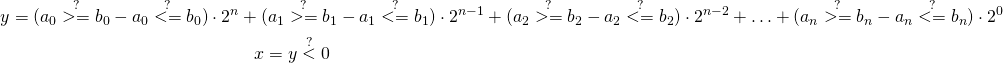OK, some black magic. Let’s untangle it.

We compare each pair of vectors’ elements. For every pair we ask whether element from vectoris greater or equal than element in vectorand subtract flag whether it is less or equal. This results in extended binary solution (minus one or zero or one). Next, we multiply this binary flag by some constant power of two. We want to do it in a way which allows us to come out with final solution just by checking the sign of the sum.

Let’s see a simple example for the following data:The idea here is: for every element we want to have positive positive term when element in vectoris greater than element in vector, zero term when they are equal, and negative term when element in vectoris less than element in vector. We achieve this by performing two comparisons: is greater or equal, and is less or equal. In the first case (element inis greater than element in), we get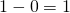. In second case we get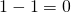, in third case we get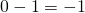.

Next, we want to get just one number representing the whole vector comparison. Since we compare numbers from left to right (see the definition of lexicographical “less than”), we multiply numbers by consecutive powers of two starting from the greatest one so the preceding terms are not “neutralized” by the next ones. Takie this example: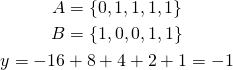Even though only first one element is lower and all the others are greater in vectorthan in vector, the total value is still negative, thanks to powers of two.

How do we perform other comparisons? It is pretty simple: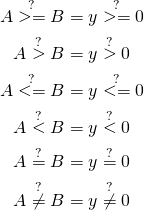# Longer vectors

We know how to compare vectors up to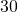elements but how to compare longer ones? Well, the trick is: let’s do the same again.

Imagine that we have vectors with hundreds of elements. We take firstelements and compare them lexicographically. We get one number which is negative, positive, or zero. The number represents relation of firstelements ofcompared lexicographically with firstelements of. We can now take nextelements and compare them in the same way. Moving forward we finally get the following vector: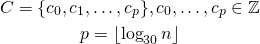This is another vector representing packages ofelements ofcompared to. We can now compare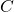with vector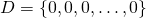of the same length.

Why does it work? Well, if firstelements ofare lexicographically lower thanelements of,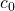will be negative so next comparison will still work well since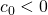. If firstelements are equal,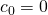so next batch ofelements will have a chance to change the comparison result. If first elements are greater then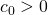which will determine the result.

We can recursively compare smaller and smaller vectors and finally end with just one number representing the solution. Finally, by comparing its sign we will be able to determine the final result.

# Summary

As we can see, we are able to compare vectors much faster with hashing approach than by using naive one.